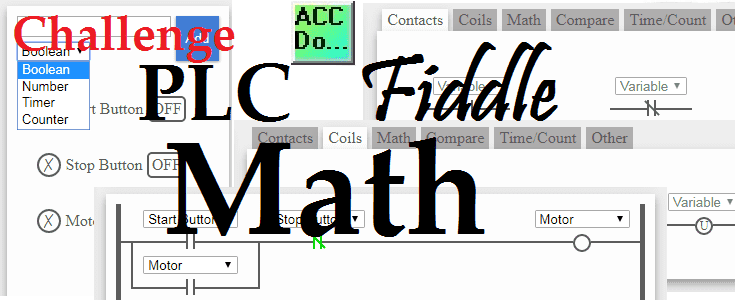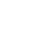# PLC Fiddle Math Challenge Examples

PLC programming will often involve math equations that need to be solved. Every manufacturer of programmable logic controllers will include math logic for you to use in your programs. Numbering systems play an important role. You can usually perform math using BCD (Binary Coded Decimal), Binary or Floating Point. Here is a link that will review the numbering systems in the PLC:
What everyone ought to know about PLC numbering systemsPLC Fiddles provides four basic BCD math and a move function for our circuits. Add, Subtract, Multiply Divide and Move. We will look at these math instructions and show you the parameters required to program them. Our math challenges will help you gain a good understanding of how we can use math in the PLC. Trying these challenges with PLC Fiddle is fun and will help you with PLC programming.
Let’s get started.

Previously we discussed the free virtual PLC simulator called PLC Fiddle and created some challenges. These challenges are ladder logic that you can solve in your browser.
PLC Fiddle Online Editor and Simulator in your BrowserVideo
AND Gate Ladder Logic Challenge Solution
OR Gate Ladder Logic Challenge Solution
NAND Gate Ladder Logic Challenge Solution
NOR Gate Ladder Logic Challenge Solution
EXOR Gate Ladder Logic Challenge Solution
EXNOR Gate Ladder Logic Challenge Solution
Timer Challenge
On-Delay Timer Challenge Solution
Off-Delay Timer Challenge Solution
Pulse Output Timer Challenge Solution
Counter Challenge
Motor Starter Service Counter Challenge Solution
Count Down Challenge Solution
Hour Minute Second Counter Challenge Solution

##### PLC Fiddle Math Variables

PLC Fiddle will use variables to perform our math operations. Variables are on the left side of our browser.The variables can be any of the following:
Number: This is a constant that we can add to our parameters.Timer.PRE – Timer Preset Value
Timer.ACC – Timer Accumulated Value (Current Value)
Counter.PRE – Counter Preset Value
Counter.ACC – Counter Accumulated Value (Current Value)
Note:
See the previous timer and counter challenges for the operation of the accumulated and preset values.
All variables are in BCD in PLC Fiddle. This makes the learning easier.

##### PLC Fiddle Math InstructionsEvery math instruction will use variables. Set the variables before you place the instruction in the work area of PLC Fiddle. You can then select the variables for each of the instructions.
ADD ( A + B = C )
This will add the first variable to the second variable and store the result in the third variable.
SUBTRACT ( A – B = C )
This will subtract the second variable from the first and store the result in the third variable.
MULTIPLY ( A * B = C )
This will multiply the first and second variables and store the result in the third.
DIVIDE ( A / B = C )
The first variable is divided by the second. The result is placed in the third variable.
MOVE
This instruction will move the first variable into the second variable. It is more like a copy because the original variable does not change its number because of the move instruction.

##### Occupancy Count Challenge – PLC Fiddle Math

Determine the number of people in a room. We have an entrance sensor for people entering the room and an exit sensor for people exiting the room. The number of people in the room will be shown in a variable called Occupancy.
https://www.plcfiddle.com/challenge/0cdd0e1b-859b-43a1-b107-44c9893bdddd
When you have finished the Occupancy Count Challenge, click the Check button to test the circuit.

Watch on YouTube: PLC Fiddle Occupancy Count Challenge Solution##### Degree F and C Challenge – PLC Fiddle Math

We have two given variables labeled Temperature Fahrenheit and Temperature Celsius. When the When Convert C input is on the Temperature Fahrenheit is converted to Celsius and the result is placed in a variable called Converted to Celsius.
When Convert F input is on the Temperature Celsius is converted to Fahrenheit and the result is placed in a variable called Converted to Fahrenheit.
Hint: C =(F – 32) / 1.8 F = C * 1.8 + 32
https://www.plcfiddle.com/challenge/2284a381-b046-4e88-ac74-4699fd7de538
When you are finished the Degree F and C Challenge, click the Check button to test the circuit.

Watch on YouTube : PLC Fiddle Degree F and C Challenge Solution##### Determine the Scan Rate of PLC Fiddle – PLC Fiddle Math

The following posts talk about Scan Rates of the PLC:
Who else wants to know how a PLC scans?
Understanding the PLC program scan.
The challenge is to determine the scan rate of this online simulator. The Scan Rate per Minute variable will be the output and will be updated every 15 seconds.
https://www.plcfiddle.com/challenge/ab949b6a-c933-4b63-9bb2-76beaa29230b
When you are finished the PLC Fiddle Scan Rate Challenge, click the Check button to test the circuit.

Watch on YouTube : Determine the Scan Rate of PLC Fiddle SolutionMath is an important part of PLC programming. I hope you found the above math challenges fun to solve using PLC Fiddle. It demonstrates the basic principles of programming without the investment of equipment. Next time we will look at the compare instruction challenge applications.

Thank you,
Garry

If you’re like most of my readers, you’re committed to learning about technology. Numbering systems used in PLC’s are not difficult to learn and understand. We will walk through the numbering systems used in PLCs. This includes Bits, Decimal, Hexadecimal, ASCII and Floating Point.

To get this free article, subscribe to my free email newsletter.

Submitting...
{{message}}

Use the information to inform other people how numbering systems work. Sign up now.## 2 thoughts on “PLC Fiddle Math Challenge Examples”

1.Wijaya says:

Sorry sir, what is the function of the circuit to determine the scan rate? I know the process but I don’t understand its function. Thanks.

1.garrys says:

Hi Wijaya,
This circuit will use the math instructions to determine the scan rate of the online PLC simulator.
The scan rate is explained in the following post:
https://accautomation.ca/understanding-the-plc-program-scan/
I hope this helps you out.
Regards,
Garry